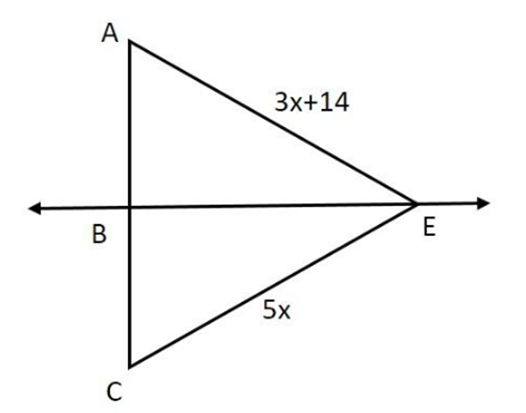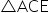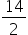Maths-
General
Easy

Question

# 𝐵𝐸 is the perpendicular bisector of 𝐴𝐶. Find 𝐶𝐸.Hint:

## The correct answer is: Hence, CE = 35 units.

### Answer: Step by step explanation:  Given: AE = 3x + 14.CE = 5xBE is perpendicular bisector at AC. Step 1: InBE is perpendicular bisector.E is point on BE.So, according to perpendicular bisector theorem,AE = CE3x + 14 = 5x14 = 5x – 3x14 = 2x= xx = 7 Step 2: Put x = 7 in 5xCE = 5xCE = 5(7)CE = 35 units. Final Answer:  Hence, CE = 35 units.#### With Turito Foundation.#### Get an Expert Advice From Turito.